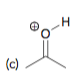# Problem: Write an equation for the proton transfer reaction that occurs when each of the following acids reacts with water. In each case, draw curved arrows that show the mechanism of the proton transfer:

###### FREE Expert Solution
87% (125 ratings)
###### Problem Details

Write an equation for the proton transfer reaction that occurs when each of the following acids reacts with water. In each case, draw curved arrows that show the mechanism of the proton transfer:What scientific concept do you need to know in order to solve this problem?

Our tutors have indicated that to solve this problem you will need to apply the Acid Base Equilibrium concept. You can view video lessons to learn Acid Base Equilibrium. Or if you need more Acid Base Equilibrium practice, you can also practice Acid Base Equilibrium practice problems.

What is the difficulty of this problem?

Our tutors rated the difficulty ofWrite an equation for the proton transfer reaction that occu...as low difficulty.

How long does this problem take to solve?

Our expert Organic tutor, Jonathan took undefined 46 seconds to solve this problem. You can follow their steps in the video explanation above.

What textbook is this problem found in?

Our data indicates that this problem or a close variation was asked in Organic Chemistry - Klein 1st Edition. You can also practice Organic Chemistry - Klein 1st Edition practice problems.